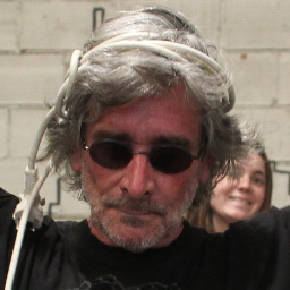Bibliothèque de traitements d'images en virgule flottante. http://la.buvette.org/photos/cumul/
You can not select more than 25 topics Topics must start with a letter or number, can include dashes ('-') and can be up to 35 characters long.

#### 48 lines 550 B Raw Normal View History Unescape Escape2 years ago ```#!/bin/bash ``` ``` ``` `PI=" 3.141592654 "`2 years ago ``` ``` `OUT="cos01.tex"`2 years ago ``` ``` `gnuplot << __EOF__` ``` ``` `set term latex` `set output "\$OUT"`2 years ago `set title "COS01"`2 years ago `set xlabel "input value"` ``` ``` `set ylabel '\rotatebox{90}{corrected value}' ` ``` ``` `set grid`2 years ago ``` ``` ```plot \ ``` ``` [0:1] [0:1] \ ``` ``` (0.5 - 0.5 * cos(x*\$PI)) \ ``` ` with line` ``` ``` `__EOF__` ``` ```2 years ago `wc \$OUT` ``` ``` `OUT="cos010.tex"` ``` ``` `gnuplot << __EOF__` ``` ``` `set term latex` `set output "\$OUT"` `set title "COS010"` `set xlabel "input value"` ``` ``` `set ylabel '\rotatebox{90}{corrected value}' ` ``` ``` `set grid` ``` ``` ```plot \ ``` ``` [0:1] [0:1] \ ``` ``` (0.5 - 0.5 * cos(x*\$PI*2)) \ ``` ` with line` ``` ``` `__EOF__` ``` ``` `wc \$OUT`